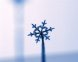# Growth

Pronunciation: /groʊθ/ Explain

Growth is an increase in quantity. When one says, "I've grown one inch in the last month," one is stating that their height has increased by one inch. Mathematicians also use the term negative growth to mean a decrease in quantity.

Mathematics is often used to study and predict growth. Models of growth that are often used by mathematicians are arithmetic growth, geometric growth and exponential growth.

### Arithmetic GrowthFigure 2: Snow crystal growth.Click on picture to see movie.Courtesy SnowCrystals.com.

Arithmetic growth occurs at a constant rate each time period. Arithmetic growth is also called constant growth and linear growth. For example, if a crystal grows in length 1 inch every year the constant rate of growth is 1 inch/year. The crystal grows 1 inch the first year, and 1 inch the second year, and so on.

YearCrystal Size
02 inches
13 inches
24 inches
35 inches
46 inches
57 inches
Table 1: Arithmetic growth of a crystal.

The general formula for arithmetic growth is y = ax + i. a represents the growth rate, and i represents the initial quantity. Using the data from Table 1, the formula for this crystal growth is y = x + 2.

Note that the equation is a linear equation in slope-intercept form (y = mx + b). When we graph arithmetic growth, the result is a line where the growth rate is the slope, and the initial quantity is the y-intercept.Figure 1: Graph showingarithmetic growth

### Geometric growth

Geometric growth occurs at an increasing or decreasing rate each time period. The general formula for geometric growth is y = a · tb where y is the quantity at time t, a is the initial quantity and b is the growth rate.

### Exponential growth

For more on exponential growth, see Exponential Function.

1. McAdams, David E.. All Math Words Dictionary, growth. 2nd Classroom edition 20150108-4799968. pg 88. Life is a Story Problem LLC. January 8, 2015. Buy the book
2. Linda Almgren Kime, Judy Clark, Beverly K. Michael. Explorations in College Algebra. 4th edition. pp 266-276,298-309. Wiley. November 16, 2007. Last Accessed 7/11/2018. Buy the book

• McAdams, David E.. Exponential Function. allmathwords.org. All Math Words Encyclopedia. Life is a Story Problem LLC. 2/8/2010. https://www.allmathwords.org/en/e/exponentialfunction.html.

McAdams, David E. Growth. 4/21/2019. All Math Words Encyclopedia. Life is a Story Problem LLC. https://www.allmathwords.org/en/g/growth.html.

### Revision History

4/21/2019: Updated equations and expressions to new format. (McAdams, David E.)
12/21/2018: Reviewed and corrected IPA pronunication. (McAdams, David E.)